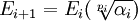## Sunday, October 19, 2008

### Abel-Ruffini Proof: Field Extensions

When Niels Abel prepared his impossibility proof, he used the vocabulary of the mathematics of his time. As a result of his work and the work done by Evariste Galois, the very language of mathematics changed. In 1995, Michael I. Rosen wrote an article for The American Mathematical Monthly that explained the ideas of Abel's proof in terms of modern ideas.

In today's blog, I will review the reinterpretation of Abel's proof in terms of modern mathematical concepts. The content in today's blog is taken from Michael Rosen's article.

The content in today's blog, assumes that you feel comfortable with the idea of fields. For review of this concept, start here.

Definition 1: subfield

A set B is a subfield of a set A if both A,B are fields and B is a subset of A.

Definition 2: field extension

A field A is a field extension of a field B if and only if B is a subfield of A.

Definition 3: splitting field over k

A field F is a splitting field over k for a polynomial f(x) if and only if F is a field extension of k and f(x) can be split into linear factors over F.

Definition 4: a radical tower over k

E/k is said to be a radical tower over k if there is a series of intermediate fields such that:

k = E0 ⊂ E1 ⊂ ... ⊂ Em-1 ⊂ Em = E

such that for each 0 ≤ i ≤ m:where pi is a prime and αi ∈ Ei

An equation f(x)=0 is solvable by radicals if and only if there is a radical tower E/k such that F is a splitting field over k and F ⊂ E.

We now state Abel's proof:

Theorem: Abel's Impossibility Proof

Let f(x) = xn - a1xn-1 + ... + (-1)nan be the general equation of degree n.

If n ≥ 5, then this equation is not solvable in radical.

Proof:

(1) An equation f(x)=0 is solvable by radicals if and only if there is a radical tower E/k such that F is a splitting field over k and F ⊂ E. [Definition 5 above]

(2) If F is contained in a radical tower over k, then F/k is itself a radical tower. [see Theorem here for proof]

(3) If n ≥ 5 and F is a splitting field, then F/k is not a radical tower. [See Theorem, here for proof]

(4) Therefore, F is not contained in a radical tower over k.

QED

References